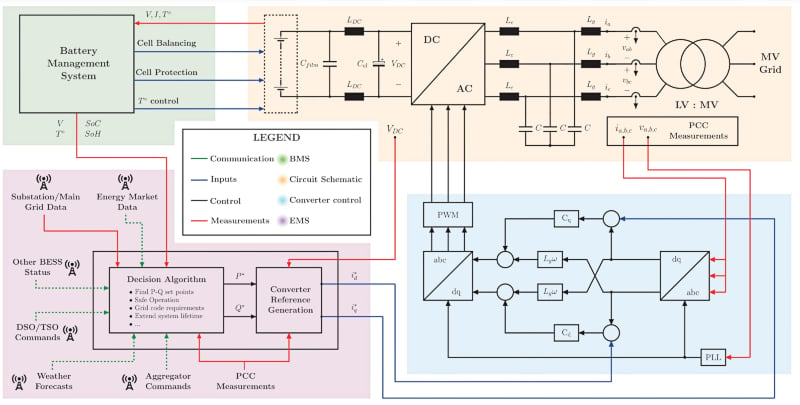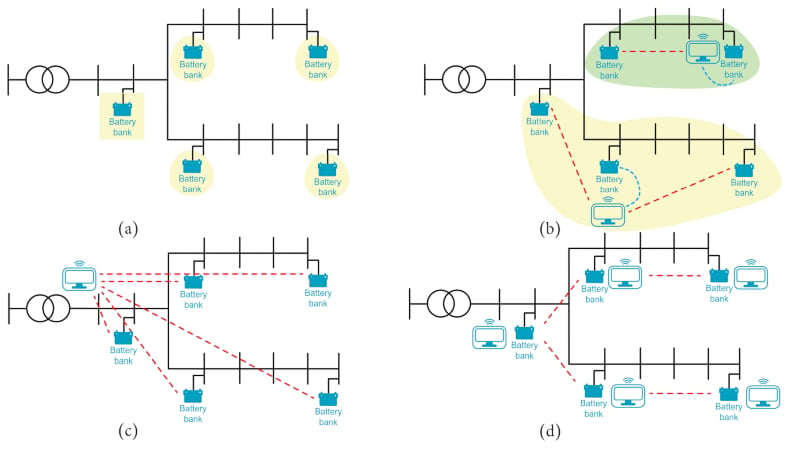Technical Article

# BESS Sizing and Placement in a Distribution Network

April 21, 2023 by Rakesh Kumar

## This article examines methods for sizing and placing battery energy storage systems in a distribution network.

The latest developments in the electricity industry encourage a high proportion of renewable energy sources. Due to their uncontrollable nature, these loads have introduced new challenges to distribution networks, making it more difficult for distribution system operators to ensure safe and dependable grid operation. Battery energy storage systems (BESSes) offer potential solutions for minimizing the effects of the new demands.### Battery Energy Storage System Sizing and Location

Several variables must be defined to solve the problem of how to best size and place storage systems in a distribution network. These are the solving method, the performance metric for the best evaluation, the battery technology and modeling, and the test network where the studies will be done.

Mathematical programming and heuristic methods are two ways to solve this problem. The difference between mathematical programming and heuristic methods is in how the optimization problem is set up and solved. Other approaches, such as analytical methods, work well in small networks but require too much computing power in complex situations.

#### Mathematical Programming

In mathematical programming, the problem is described by a set of equations. Different ways to solve the problem can be used depending on the properties of these equations. These can be linear, semi-definite, or second-order cone problems. There are a lot of commercial solvers that can solve convex optimization problems, and these have been used a lot in power system applications because they work well.

#### Heuristic Methods

Heuristic methods can be used for multi-objective optimization because they do not need exact mathematical formulations. The most common way to use these algorithms is to use artificial intelligence to figure out the size and location of the storage systems and then use classic optimal power flow algorithms to figure out which candidates are the best. Heuristic methods have been changed in many ways.

However, particle swarm optimization (PSO) and genetic algorithms (GA) are two of the most common algorithms. PSO has been used to solve some of the most common problems with power systems, such as load flow, voltage control, and economic dispatch. Because of how the size and placement of energy storage work, PSO and GA are good options for an optimization algorithm.

#### Mathematical Programming vs. Heuristic Methods

Table 1 compares the two types of optimization techniques. When convex programs are used, the problem must be stated clearly. In this way, heuristic methods are more flexible, but the solutions are less accurate. There is no guarantee these algorithms will reach the global optimum, and they may get stuck in a local optimum.

##### Table 1. Mathematical Programming vs. Heuristic Methods
 Mathematical Programming Heuristic Methods Pros Strong in finding global optimum     Mature in power systems     Good computational performance Flexible in solving all problems     Avoid complex derivations     Less computationally demanding Cons Convergence difficulties     Explicit mathematical formulation required May converge to a local optimum     Less accuracy

In mathematical programming, criteria like where storage is placed to minimize the virtual operation costs, the costs of buying energy, and the costs of the system, losses, and the flow of power at the substation are looked into. Heuristic methods have been used to find the optimal storage capacity. It minimizes losses and voltage violations after finding the best location through loss sensitivity analysis to solve the grid expansion plan to lower total system costs. It is also used to reduce storage capital costs when a BESS is used to prevent overvoltages and avoid network congestion.

The planning problem is usually simplified by looking at it discretely, where the storage size is thought of in steps of 10 or 100 kWh, sequentially, where first the best location is found, and then the battery capacity is determined. These strategies might make the problem easier to set up, but the answer may be less accurate. Another interesting way to set up multiple storage units is to divide the network into different clusters and determine the best place for each unit within each cluster.

Several electrochemical technologies, like lithium-ion, vanadium redox, NaS, and lead-acid, have been thought about. However, too simple a model can lead to big mistakes in estimating the lifetime and aging. Studies show that non-optimal BESS scheduling hurts the BESS lifetime when a simplified model is used. The amount of time needed to do the calculations went up a lot, but it was still fine.

### BESS Management and Operation

Figure 1 shows the main parts of a battery energy storage system that are necessary for it to work. The battery management system (BMS) takes measurements from the electrochemical storage and balances the voltage of the cells, keeping them from overloading and reducing temperature differences so the cells age evenly. The BMS determines how charged the battery is and how healthy it is. It sends this information to the energy management system (EMS), which runs and protects the storage system.

As shown in Figure 1, the EMS gets information from the BMS about the battery parameters and other sources like electrical measurements at the point of common coupling (PCC), weather forecasts, energy market data, and commands from distribution system operators, transmission system operators, and aggregators.

With this information, the EMS's built-in decision algorithm finds the P-Q set points of the storage systems. These are digitally changed into the reference values for the DC-AC converter and sent to the control board that runs the system. In Figure 1, the light blue block shows a current control scheme for the storage system based on the dq frame.##### Figure 1. Battery energy storage system circuit schematic and main components. Image used courtesy of IEEE Open Journal of the Industrial Electronics Society

The EMS is also in charge of scheduling BESS operations and coordinating the different storage units when there is more than one BESS. Each BESS unit could have an independent EMS that manages the storage system based on local measurements, as shown in Figure 2(a). Alternatively, there could be a supervisory unit that manages a portion of the grid, as shown in Figure 2(b), or the whole network, as shown in Figure 2(c), or each unit could have a decision-making algorithm that can talk to neighboring units, as shown in Figure 2(d). These management methods are called local, decentralized, centralized, and distributed.##### Figure 2. Examples of BESS management strategies: a) Local control, b) Decentralized control, c) Centralized control, and d) Distributed control. Image used courtesy of IEEE Open Journal of the Industrial Electronics Society

When making a BESS control system, the biggest problems are the communication infrastructure, decision-making algorithm, and dealing with the uncertainty in the data and mistakes in the forecasts. The infrastructure for communication can be expensive, especially in grids that cover a large area. For a control strategy to work well, there may be strict reliability, latency, and data rate requirements.

### Takeaways of Battery Energy Storage System Sizing and Location

This article has discussed BESS sizing, location in the distribution network, management, and operation. Some of the takeaways follow.

• BESS sizing and placement issues in the distribution network can be resolved with mathematical programming and heuristic techniques.
• A set of equations describes the issue in mathematical programming. Some common terms for it include linear problem, semi-definite problem, and second-order cone problem.
• Heuristic methods can be used for multi-objective optimization because no precise mathematical formulations are required. Among the most popular algorithms are GA and PSO.
• Research shows that, even with a simplified model, non-optimal BESS scheduling reduces the BESS's useful lifetime.
• The BMS is in charge of balancing the cell voltage, preventing them from being overloaded, and minimizing temperature differences for uniform cell aging.
• When there are multiple BESS, the EMS is in charge of planning BESS operations and coordinating the various storage facilities.
• Putting in place a reliable and cost-effective communication infrastructure for BESS can be challenging and costly, especially for wide-area grids.
• For a BESS control strategy to be effective, stringent reliability, latency, and data rate requirements may be necessary.

This post is based on an IEEE Open Journal of the Industrial Electronics Society research article.